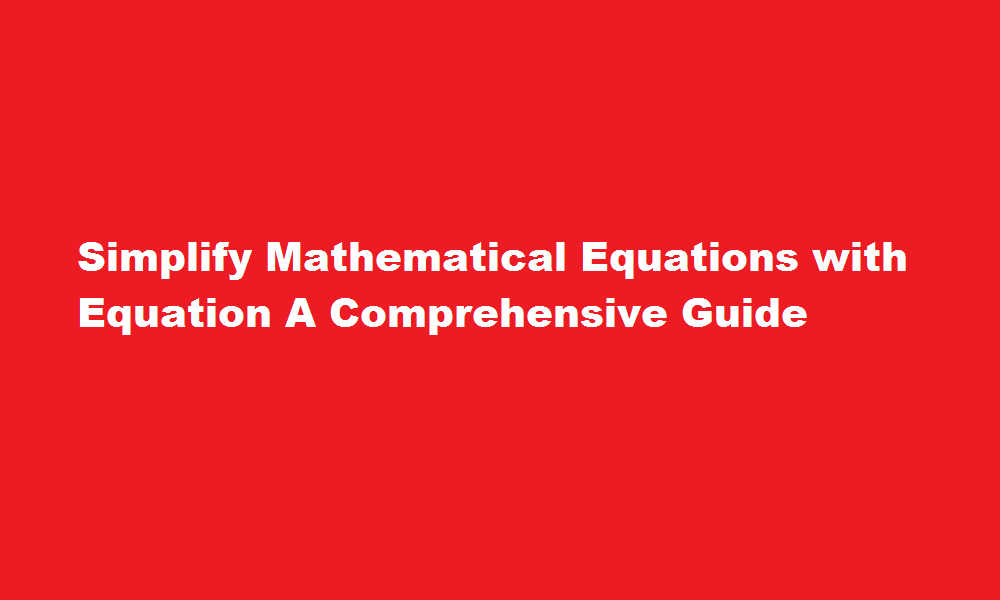September 26, 2023
[stock-market-ticker]

# Simplify Mathematical Equations with Equation A Comprehensive GuideContents

## Introduction

Mathematics is an integral part of our lives, and technology has greatly enhanced our ability to solve complex equations efficiently. EquatIO is a powerful tool that enables users to create, manipulate, and solve mathematical equations digitally. In this article, we will explore the features and functionality of EquatIO and provide a step-by-step guide on how to make the most of this versatile tool.

## Understanding EquatIO

EquatIO is a software extension designed to facilitate the creation and manipulation of mathematical equations across various digital platforms. Developed by Texthelp, EquatIO integrates seamlessly with popular applications such as Microsoft Word, Google Docs, Google Forms, and other online platforms. Its intuitive interface and extensive array of mathematical symbols make it an indispensable tool for educators, students, and professionals.

Also Read  Cracking The UPSC Interview Strategies for Success

## Installation and Setup

To begin using EquatIO, you’ll need to install the appropriate extension for your preferred platform. EquatIO is available as a browser extension for Chrome, Firefox, and Edge, as well as a desktop application for Windows and macOS. After installation, you may need to sign in using your Google or Microsoft account to activate the tool fully. Once you’re set up, EquatIO will be ready to use in your chosen application.

## Creating Mathematical Equations

EquatIO provides several methods for creating equations. You can either use the user-friendly equation editor with its extensive library of symbols, or you can utilize the handwriting recognition feature to write equations directly using a mouse or touchscreen. EquatIO recognizes and converts handwritten equations into digital format, ensuring accuracy and ease of use. Additionally, EquatIO allows you to dictate equations using voice input, making it accessible to users with limited dexterity.

## Manipulating Equations

EquatIO’s versatility extends beyond equation creation. Once you’ve created an equation, you can easily edit, rearrange, and modify it to suit your needs. EquatIO recognizes mathematical structures and allows you to manipulate individual elements within an equation, such as fractions, exponents, and radicals. You can resize and rearrange equations, change fonts and colors, and even convert handwritten equations into more readable typeset format. These features make it effortless to fine-tune equations and express mathematical concepts accurately.

Also Read  A Step-by-Step Guide to Submitting IGNOU Assignments

## Integrating Equations into Documents and Presentations

EquatIO’s seamless integration with popular word processors and presentation software is a significant advantage. You can insert equations directly into your documents, worksheets, or presentations with a simple click or keystroke. EquatIO ensures that equations are properly formatted and aligned, preserving their clarity and readability. Furthermore, equations created with EquatIO can be exported as images or shared as interactive components that allow collaborators to interact with and solve equations directly.

### Can students use Equatio?

Equatio is a powerful equation editor that makes it easy to create digital, accessible math. It saves time, lowers stress and eliminates frustration. It makes math and science more accessible and engaging for every student.

Also Read   Removing Ink Stains from Shoes Effective Techniques and Tips

### Does equatio work in words?

Equatio® for Windows integrates easily with Microsoft Word*. It lets you add math formulas and other expressions into documents with a click.

## Conclusion

EquatIO is a versatile tool that simplifies the creation, manipulation, and integration of mathematical equations into digital documents. Whether you’re an educator preparing lesson materials, a student taking notes, or a professional presenting complex data, EquatIO empowers you to express mathematical ideas with clarity and precision. By combining user-friendly interfaces, advanced recognition capabilities, and seamless integration, EquatIO enhances the accessibility and efficiency of working with mathematical equations. Embrace the power of EquatIO, and unlock new possibilities in your mathematical journey.